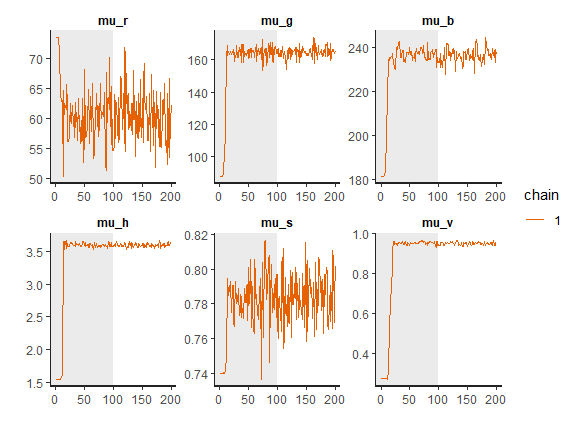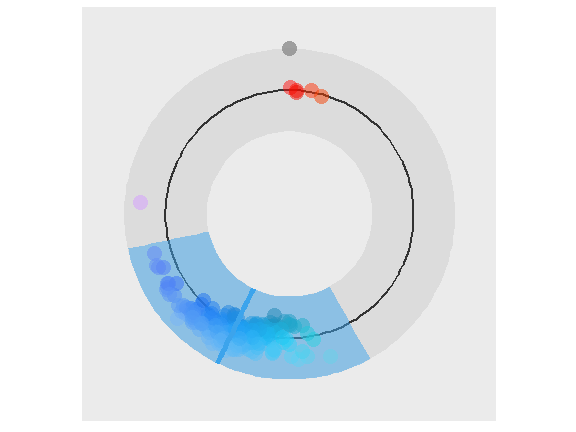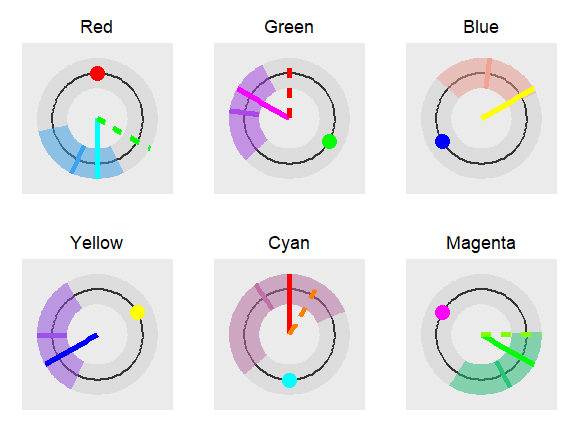# Introduction

In the afterimages task participants were asked to ﬁx their gaze on a ﬁxation point in the middle of the computer screen. Stimulus – a colored rectangle – was then shown above the ﬁxation point. After 20 seconds the rectangle disappeared and a color palette was shown on the right-hand side of the screen. Participants were asked to keep their gaze on the ﬁxation point while using the mouse to select the color that best matched the color of the afterimage that appeared above the ﬁxation point. To help select the correct color, a rectangle of the same size as the adapting stimuli was shown below the ﬁxation point in the color currently under the mouse cursor. Participants conﬁrmed their selection by pressing a mouse button when they were satisfied that color of the rectangle below the fixation point matched the color of the afterimage experienced above the fixation point. For each trial the color of the stimulus rectangle, the subject’s response in RGB and the subject’s response time were recorded. The goal of this study was to determine which of the two color coding mechanisms (trichromatic or opponent-process) better explains the perceived color of the afterimages. We used six differently colored rectangles: red, green, blue, cyan, magenta, yellow.

We start our analysis by loading the experiment and stimuli data. The experiment data include subject index, reaction time, response in RGB format, stimuli name (e.g blue) and stimuli values in RGB and HSV. The stimuli data set includes only the information about stimuli (names, RGB and HSV values).

# libs
library(bayes4psy)
library(cowplot)
library(dplyr)
library(ggplot2)

data_all <- after_images

stimuli <- after_images_stimuli

Once we load required libraries and thata we can start fitting the Bayesian color models. Below is a detailed example of fitting the Bayesian color model for red color stimuli. Note here that, due to vignette limitations, all fits are built using only one chain, using more chains in parallel is usually more efficient. Also to increase the building speed of vignettes we greatly reduced the amount of iterations, use an appropriate amount of iterations when executing actual analyses!

# prepare data
data_red <- data_all %>% filter(stimuli == "red")
data_red <- data.frame(r=data_red$r, g=data_red$g,
b=data_red$b) # fit fit_red <- b_color(colors=data_red, chains=1, iter=200, warmup=100) ## ## SAMPLING FOR MODEL 'color' NOW (CHAIN 1). ## Chain 1: ## Chain 1: Gradient evaluation took 6.7e-05 seconds ## Chain 1: 1000 transitions using 10 leapfrog steps per transition would take 0.67 seconds. ## Chain 1: Adjust your expectations accordingly! ## Chain 1: ## Chain 1: ## Chain 1: WARNING: There aren't enough warmup iterations to fit the ## Chain 1: three stages of adaptation as currently configured. ## Chain 1: Reducing each adaptation stage to 15%/75%/10% of ## Chain 1: the given number of warmup iterations: ## Chain 1: init_buffer = 15 ## Chain 1: adapt_window = 75 ## Chain 1: term_buffer = 10 ## Chain 1: ## Chain 1: Iteration: 1 / 200 [ 0%] (Warmup) ## Chain 1: Iteration: 20 / 200 [ 10%] (Warmup) ## Chain 1: Iteration: 40 / 200 [ 20%] (Warmup) ## Chain 1: Iteration: 60 / 200 [ 30%] (Warmup) ## Chain 1: Iteration: 80 / 200 [ 40%] (Warmup) ## Chain 1: Iteration: 100 / 200 [ 50%] (Warmup) ## Chain 1: Iteration: 101 / 200 [ 50%] (Sampling) ## Chain 1: Iteration: 120 / 200 [ 60%] (Sampling) ## Chain 1: Iteration: 140 / 200 [ 70%] (Sampling) ## Chain 1: Iteration: 160 / 200 [ 80%] (Sampling) ## Chain 1: Iteration: 180 / 200 [ 90%] (Sampling) ## Chain 1: Iteration: 200 / 200 [100%] (Sampling) ## Chain 1: ## Chain 1: Elapsed Time: 0.044 seconds (Warm-up) ## Chain 1: 0.037 seconds (Sampling) ## Chain 1: 0.081 seconds (Total) ## Chain 1: # inspect plot_trace(fit_red)plot_hsv(fit_red)# the command below is commented out for the sake of brevity #print(fit_red) The function plot_hsv was developed specially for the color model. Input data points are visualized with circles, mean of the fit is visualized with a solid line and the 95% HDI of the underlying distribution is visualized as a colored band. If we are satisfied with the fit we repeat the whole process five more times for the remaining five colors of stimuli. For brevity only code for fitting the model is executed here, the inspections of fits are ommited. # green data_green <- data_all %>% filter(stimuli == "green") data_green <- data.frame(r=data_green$r,
g=data_green$g, b=data_green$b)
fit_green <- b_color(colors=data_green, chains=1, iter=200, warmup=100)

# blue
data_blue <- data_all %>% filter(stimuli == "blue")
data_blue <- data.frame(r=data_blue$r, g=data_blue$g,
b=data_blue$b) fit_blue <- b_color(colors=data_blue, chains=1, iter=200, warmup=100) # yellow data_yellow <- data_all %>% filter(stimuli == "yellow") data_yellow <- data.frame(r=data_yellow$r,
g=data_yellow$g, b=data_yellow$b)
fit_yellow <- b_color(colors=data_yellow, chains=1, iter=200, warmup=100)

# cyan
data_cyan <- data_all %>% filter(stimuli == "cyan")
data_cyan <- data.frame(r=data_cyan$r, g=data_cyan$g,
b=data_cyan$b) fit_cyan <- b_color(colors=data_cyan, chains=1, iter=200, warmup=100) # magenta data_magenta <- data_all %>% filter(stimuli == "magenta") data_magenta <- data.frame(r=data_magenta$r,
g=data_magenta$g, b=data_magenta$b)
fit_magenta <- b_color(colors=data_magenta, chains=1, iter=200, warmup=100)

We start the analysis by loading data about the colors predicted by the trichromatic or the opponent-process theory. We can then use the plot_distributions_hsv function of the Bayesian color model to produce a visualization of the accuracy of both color coding mechanisms predictions for each stimuli independently. Each graph visualizes the fitted distribution, displayed stimuli and responses predicted by the trichromatic and opponent-process coding. This additional information can be added to the visualization via annotation points and lines.

# load theory predictions
trichromatic <- after_images_trichromatic
opponent_process <- after_images_opponent_process

# red
stimulus <- "red"
lines <- list()
lines[] <- c(trichromatic[trichromatic$stimuli == stimulus, ]$h,
trichromatic[trichromatic$stimuli == stimulus, ]$s,
trichromatic[trichromatic$stimuli == stimulus, ]$v)
lines[] <- c(opponent_process[opponent_process$stimuli == stimulus, ]$h,
opponent_process[opponent_process$stimuli == stimulus, ]$s,
opponent_process[opponent_process$stimuli == stimulus, ]$v)

points <- list()
points[] <- c(stimuli[stimuli$stimuli == stimulus, ]$h_s,
stimuli[stimuli$stimuli == stimulus, ]$s_s,
stimuli[stimuli$stimuli == stimulus, ]$v_s)

plot_red <- plot_distributions_hsv(fit_red, points=points,
lines=lines, hsv=TRUE)

plot_red <- plot_red + ggtitle("Red") +
theme(plot.title = element_text(hjust = 0.5))

# green
stimulus <- "green"
lines <- list()
lines[] <- c(trichromatic[trichromatic$stimuli == stimulus, ]$h,
trichromatic[trichromatic$stimuli == stimulus, ]$s,
trichromatic[trichromatic$stimuli == stimulus, ]$v)
lines[] <- c(opponent_process[opponent_process$stimuli == stimulus, ]$h,
opponent_process[opponent_process$stimuli == stimulus, ]$s,
opponent_process[opponent_process$stimuli == stimulus, ]$v)

points <- list()
points[] <- c(stimuli[stimuli$stimuli == stimulus, ]$h_s,
stimuli[stimuli$stimuli == stimulus, ]$s_s,
stimuli[stimuli$stimuli == stimulus, ]$v_s)

plot_green <- plot_distributions_hsv(fit_green, points=points,
lines=lines, hsv=TRUE)
plot_green <- plot_green + ggtitle("Green") +
theme(plot.title = element_text(hjust = 0.5))

# blue
stimulus <- "blue"
lines <- list()
lines[] <- c(trichromatic[trichromatic$stimuli == stimulus, ]$h,
trichromatic[trichromatic$stimuli == stimulus, ]$s,
trichromatic[trichromatic$stimuli == stimulus, ]$v)
lines[] <- c(opponent_process[opponent_process$stimuli == stimulus, ]$h,
opponent_process[opponent_process$stimuli == stimulus, ]$s,
opponent_process[opponent_process$stimuli == stimulus, ]$v)

points <- list()
points[] <- c(stimuli[stimuli$stimuli == stimulus, ]$h_s,
stimuli[stimuli$stimuli == stimulus, ]$s_s,
stimuli[stimuli$stimuli == stimulus, ]$v_s)

plot_blue <- plot_distributions_hsv(fit_blue, points=points,
lines=lines, hsv=TRUE)
plot_blue <- plot_blue + ggtitle("Blue") +
theme(plot.title = element_text(hjust = 0.5))

# yellow
stimulus <- "yellow"
lines <- list()
lines[] <- c(trichromatic[trichromatic$stimuli == stimulus, ]$h,
trichromatic[trichromatic$stimuli == stimulus, ]$s,
trichromatic[trichromatic$stimuli == stimulus, ]$v)
lines[] <- c(opponent_process[opponent_process$stimuli == stimulus, ]$h,
opponent_process[opponent_process$stimuli == stimulus, ]$s,
opponent_process[opponent_process$stimuli == stimulus, ]$v)

points <- list()
points[] <- c(stimuli[stimuli$stimuli == stimulus, ]$h_s,
stimuli[stimuli$stimuli == stimulus, ]$s_s,
stimuli[stimuli$stimuli == stimulus, ]$v_s)

plot_yellow <- plot_distributions_hsv(fit_yellow, points=points,
lines=lines, hsv=TRUE)
plot_yellow <- plot_yellow + ggtitle("Yellow") +
theme(plot.title = element_text(hjust = 0.5))

# cyan
stimulus <- "cyan"
lines <- list()
lines[] <- c(trichromatic[trichromatic$stimuli == stimulus, ]$h,
trichromatic[trichromatic$stimuli == stimulus, ]$s,
trichromatic[trichromatic$stimuli == stimulus, ]$v)
lines[] <- c(opponent_process[opponent_process$stimuli == stimulus, ]$h,
opponent_process[opponent_process$stimuli == stimulus, ]$s,
opponent_process[opponent_process$stimuli == stimulus, ]$v)

points <- list()
points[] <- c(stimuli[stimuli$stimuli == stimulus, ]$h_s,
stimuli[stimuli$stimuli == stimulus, ]$s_s,
stimuli[stimuli$stimuli == stimulus, ]$v_s)

plot_cyan <- plot_distributions_hsv(fit_cyan, points=points,
lines=lines, hsv=TRUE)
plot_cyan <- plot_cyan + ggtitle("Cyan") +
theme(plot.title = element_text(hjust = 0.5))

# magenta
stimulus <- "magenta"
lines <- list()
lines[] <- c(trichromatic[trichromatic$stimuli == stimulus, ]$h,
trichromatic[trichromatic$stimuli == stimulus, ]$s,
trichromatic[trichromatic$stimuli == stimulus, ]$v)
lines[] <- c(opponent_process[opponent_process$stimuli == stimulus, ]$h,
opponent_process[opponent_process$stimuli == stimulus, ]$s,
opponent_process[opponent_process$stimuli == stimulus, ]$v)

points <- list()
points[] <- c(stimuli[stimuli$stimuli == stimulus, ]$h_s,
stimuli[stimuli$stimuli == stimulus, ]$s_s,
stimuli[stimuli$stimuli == stimulus, ]$v_s)

plot_magenta <- plot_distributions_hsv(fit_magenta, points=points,
lines=lines, hsv=TRUE)
plot_magenta <- plot_magenta + ggtitle("Magenta") +
theme(plot.title = element_text(hjust = 0.5))

We can then use the cowplot library to combine the plots into a single figure.

plot_grid(plot_red, plot_green, plot_blue,
plot_yellow, plot_cyan, plot_magenta,
ncol=3, nrow=2, scale=0.9)This last figure features the comparison of predictions between the thrichromatic and the oponent-process color coding. The long solid line visualizes the trichromatic color coding prediction while the dashed line visualizes the opponent-process color coding. Short solid line represents the mean hue of the fit and the the colored band the 95% HDI of the distribution underlying the fit. The small colored circle visualizes the color of the presented stimuli. In the case of blue and yellow stimuli the dashed line is not visible because both color codings predict the same outcome. The prediction based on the thrichromatic color coding seems more accurate as its prediction is always inside the 95% of the most probable subject’s responses and is always closer to the mean predicted hue than the opponent-process prediction. The opponent-process prediction is outside of the 95% of the most probable subject’s responses in cases of red and green stimuli.